Courses
Courses for Kids
Free study material
Free LIVE classes
More

# JEE Important Chapter - Atomic Structure

Get interactive courses taught by top teachers## Introduction to Atomic Structure

Last updated date: 21st Sep 2023
Total views: 144k
Views today: 3.48k

Since the time of early Indian and Greek thinkers (400 B.C.), who believed that atoms are the fundamental building blocks of the universe, the presence of atoms has been posited as matter's building blocks of matter. They claim that the current situation is unsustainable and the Subdivisions of matter would eventually give rise to atoms and these would not be divisible any further. The term 'atom' has been coined. The word 'a-tomio' is derived from the Greek word 'a-tomio', which implies 'Uncut-able' or 'non-divisible' is a term used to describe something that cannot be cut or divided. These previous concepts were. There was no way to test these theories because they just guessed experimentally. For a long time, these concepts were dormant and were resurrected by scientists in the 1990s.

On a small scale, the atomic theory of matter was originally postulated. John Dalton, a British school teacher, established a robust scientific foundation in 1808. The atom, according to Dalton's atomic theory, is the ultimate particle of matter. Dalton's atomic theory was particularly successful in explaining the laws of mass conservation, constant composition, and multiple proportions. However, it was known that objects like glass or ebonite get electrically charged when touched with silk or fur, but it failed to explain the results of many studies.

## JEE Main Chemistry Chapter-wise Solutions 2022-23

### Important Topics for Atomic Structure

• Introduction to  Atomic structure

• Developments leading to the Bohr's Model of an atom

• Quantum Mechanical Model of an atom

• Discovery of subatomic particles

• Bohr's Model for Hydrogen atom

• Stability of Completely Filled and Half Filled Subshells

• Atomic Models

• Towards Quantum Mechanical Model of an atom

### 1.  Discovery of Subatomic Particles

Experiments on electrical discharge through gases provided insight into the structure of the atom. Before we go over these findings, it's important to remember a basic law about charged particle behaviour: "Like charges repel one another, while unlike charges attract each other."

(a)  Discovery of Electron

• William Crookes investigated the electrical discharge in cathode ray discharge tubes, which are partially evacuated tubes.

• A discharge tube is a glass tube that is roughly 60cm long and has two thin metal bits called electrodes that are sealed inside. Crookes tube is the name for this type of tube. The negative electrode is referred to as the cathode, whereas the positive electrode is referred to as the anode Invisible rays emanating from the cathode and producing a greenish light behind the perforated anode on the glass wall coated with phosphorescent substance ZnS are detected when a gas confined at low pressure              (10-4 atm) in a discharge tube is subjected to a high voltage (10,000V). Cathode rays were the name given to these beams. They ionise the gases they pass through.

• When they collide with a metallic target, they emit X-rays. The material of the electrodes and the nature of the gas present in the cathode ray tube have no effect on the characteristics of cathode rays (electrons). As a result, we can conclude that electrons are the fundamental building blocks of all matter.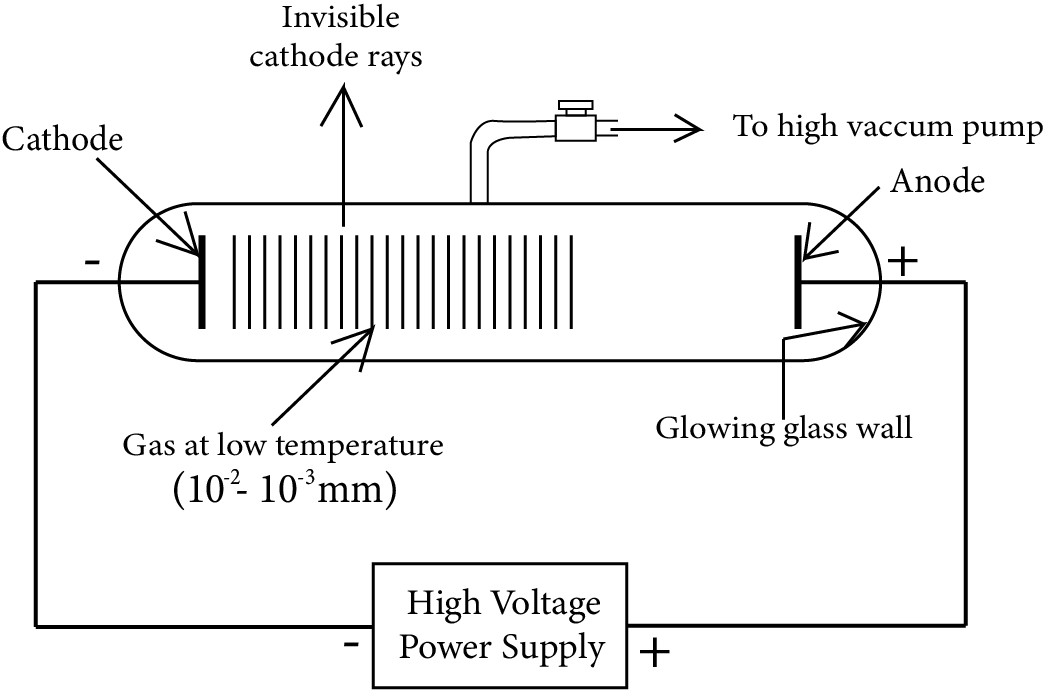(b) Charge to Mass Ratio of Electron

• J.J. Thomson used a cathode ray tube to measure the e/m ratio of electrons in 1897, employing electric and magnetic fields that were perpendicular to each other and to the path of electrons.

• In the presence of an electric or magnetic field, the extent to which electrons deviate from their path is determined by: (a) the charge on the electron, (b) the mass of the particle, and (c) the strength of the electric or magnetic field.

• The electrons are deflected to point A when merely an electric field is applied. Electrons are deflected to point C when merely a magnetic field is provided. The electrons are permitted to reach the screen at point B (the point where electrons impact in the screen) by balancing the intensities of electric or magnetic fields.

• By measuring the amount of deflections Thomson was able to calculate the value of e/m as 1.758820 × 1011C/kg.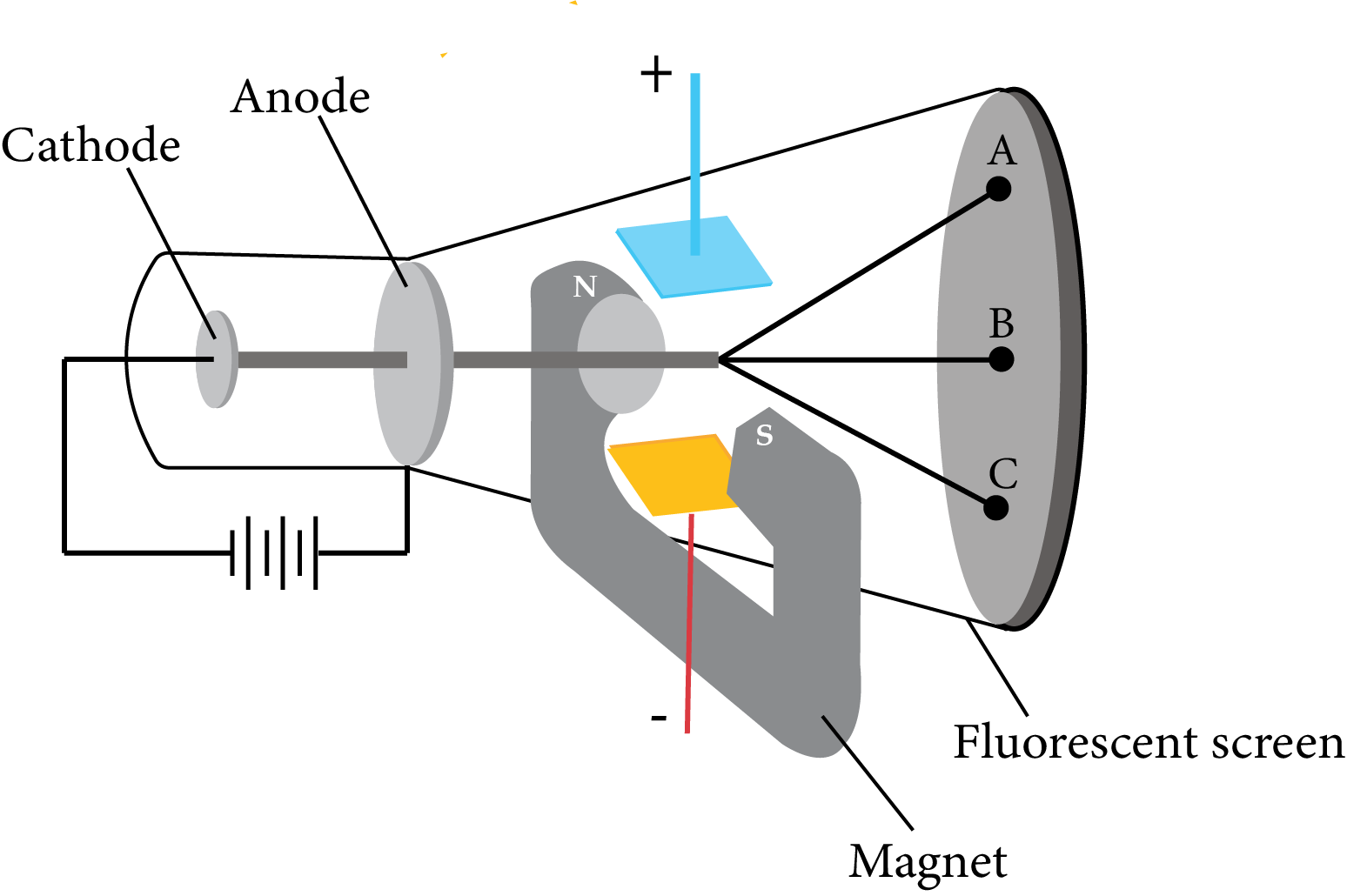(c) Charge on the Electron

• To establish the charge on the electrons, R.A Millikan invented the oil drop experiment. Oil droplets in the form of mist are created in this approach. The atomiser was permitted to enter through a small hole in the ceiling of the electrical condenser's upper plate. The declining trend through the telescope, the movement of these droplets was observed. A micrometre eyepiece is included. By calculating Millikan was able to measure the pace at which these drops fell and  a clump of oil droplets The air within the chamber was suffocating. It was ionised by running an X-ray beam through it. The electrical system Collisions with these oil droplets gave them a charge.

• Millikan discovered that the magnitude of electrical charge, q, on oil droplets is always an integral multiple of the electrical charge, e, by carefully monitoring the effects of electric field intensity on the motion of oil droplets, q = n e, where n = 1, 2, 3...

• The electron's charge is measured to be –1.6022 X 10–19C.

(d) Discovery of Protons and Neutrons

• The discovery of canal rays containing positively charged particles was made possible by an electrical discharge in a modified cathode ray tube. The following is a list of the features of these positively charged particles.

(i) Unlike cathode rays, the mass of positively charged particles is determined by the type of gas in the cathode ray tube. Simply put, these are positively charged gaseous ions.

(ii) The charge-to-mass ratio of the particles is affected by the gas from which they come.

(iii) A multiple of the fundamental unit of electrical charge is carried by some positively charged particles.

(iv) The behaviour of these particles in the magnetic or electrical field is opposite to that is observed for electron or cathode rays.

• The smallest and lightest positive ion was obtained from hydrogen and was called

proton. This positively charged particle was characterised in 1919. Later, a need was felt for the presence of electrically neutral particles as one of the constituents of atoms. These particles were discovered by Chadwick (1932) by bombarding a thin sheet of beryllium with α-particles. When electrically neutral particles having a mass slightly greater than that of protons were emitted. He named these particles as neutrons. Some of the positively charged particles carry multiple of fundamental units of electrical charge.

### 2.  Atomic Models

According to the results of the tests described in the previous sections, Dalton's indivisible atom is made up of subatomic particles with positive and negative charges. Following the discovery of subatomic particles, scientists faced several key challenges, including

• Accounting for atom stability;

• Comparing the behaviour of elements in terms of both physical and chemical properties, and

• To comprehend the origin and nature of the features of electromagnetic radiation received or emitted by atoms, and to explain the formation of various types of molecules by the combination of various atoms.

(a) Thomson Model of Atom

• Sir J. J. Thomson, the inventor of the electron, was the first to propose an atomic structure model.

(i) Electrons are found in every atom.

(ii) The entire atom is neutral. The total positive and negative charges must be the same.

He saw the atom's positive charge is evenly distributed around an atomic-sized sphere (about 10–10 m in diameter).

• The electrons are the building blocks of the universe  and these are smaller particles together carrying a negative charge, equal to the positive charge in the atom. They were studded in the atom-like plums in a pudding. The charge distribution was such that it gave the most stable arrangement. This model of the atom was often called the plum–pudding model. Also the raisin pudding model or watermelon model.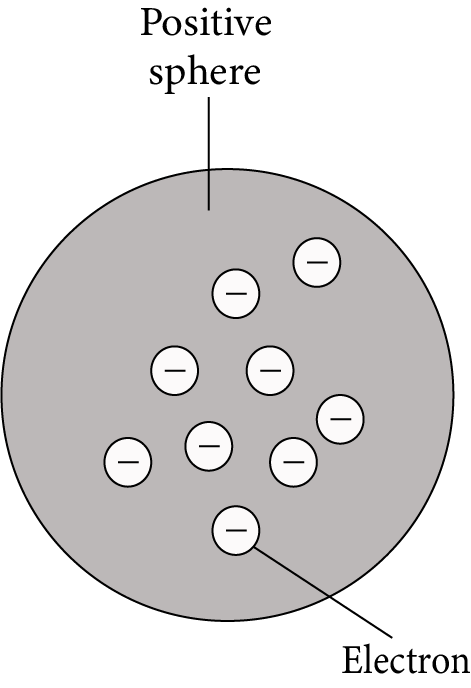(b) Rutherford’s Nuclear Model of Atom

1. At the centre of an atom lies a tiny positively charged nucleus, which is surrounded by a hollow region known as the extranuclear part.

2. Nucleons, which are made up of protons and neutrons, give the nucleus its positive charge, whereas electrons, which are found in the extranuclear region, have minimal mass and carry a negative charge.

3. Because the number of electrons in the atom equals the number of protons, it is electrically neutral. As a result, the nucleus' total positive charge is balanced by the electrons' total negative charge.

4. In the extranuclear section, electrons orbit the nucleus in circular routes known as orbits. As a result, an atom resembles the solar system, where the sun serves as the nucleus. The planets are made up of rotating electrons, and the model is called the planetary model.

5. The electrostatic force of attraction holds electrons and nuclei together.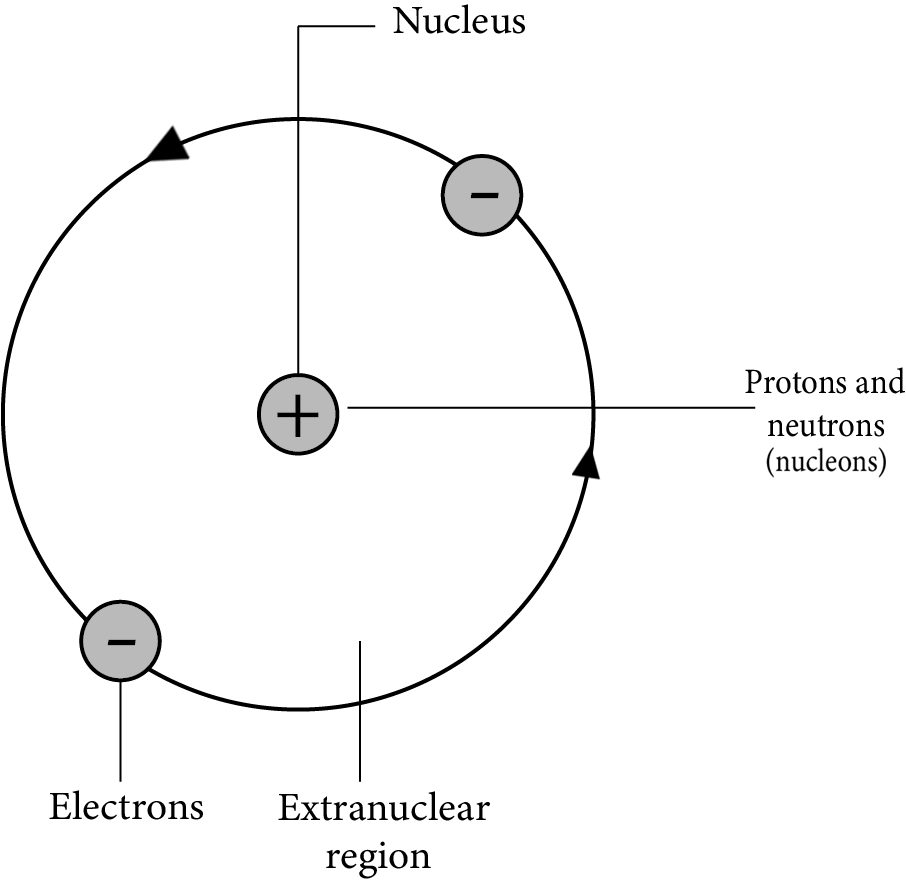Drawbacks

(i) Any charged entity in motion under the influence of attractive forces should be according to classical mechanics and these are Continuously emitting energy. If this is the case, the electron will be ejected and take a spiral path until we will reach the nucleus and in the nucleus, the structure would come crashing down. This type of behaviour is never seen.

(ii) It makes no mention of atoms' electrical structure, i.e. the distribution of electrons around the nucleus.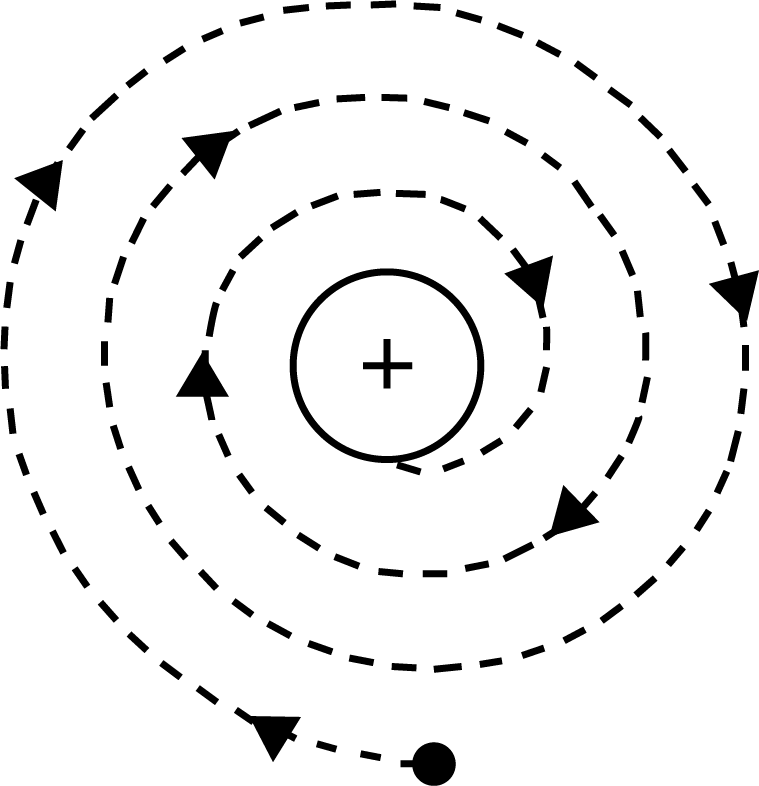(c) Atomic Number and Mass Number

• The number of unit positive charges or protons contained in the nucleus of an element's atom is equal to the element's atomic number. It also represents the neutral atom's number of electrons. Eg. The number of protons in Na is 11, hence its atomic number is 11 5.2 a large number (A)

• The nucleons are the elementary particles (protons and neutrons) that make up the nucleus of an atom.

• An atom's mass number (A) is equal to the total of its protons and neutrons. It's always a number that's a whole number. As a result, the mass number (A) equals the sum of the number of protons (Z) and the number of neutrons (n).

• The total number of nucleons is termed as mass number (A) of the atom.

• Mass number (A) = number of protons (Z) + number of neutrons (n)

(d)  Isobars and Isotopes

• Isobars are atoms that have the same mass number but a different atomic number, such as   614C and 714N.

• Isotopes are the same atomic number but different mass number atoms of the same element. The discrepancy is due to the number of neutrons present.

• The number of electrons in an atom determines its chemical characteristics. As a result, isotopes of the same element have the same chemical behaviour.

• Consider the hydrogen atom, 99.985 percent of hydrogen atoms have only one proton.

• Protium  is the name of this isotope. The remaining fraction of hydrogen atoms comprises two other isotopes: deuterium and tritium. Deuterium has one proton and one neutron, while tritium has one proton and two neutrons.

### 3. Developments Leading to the Bohr's Model of an Atom

These findings were used by Neils Bohr to improve on Rutherford's model. The formulation of Bohr's atom model was influenced by two main advances. These included (i) the dual character of electromagnetic radiation, which means that radiations have both wave and particle features.

(ii) experimental observations on atomic spectra.

(a) Wave Nature of Electromagnetic Radiation

• On a macroscopic level, James Maxwell (1870) was the first to give a full explanation of the interaction between charged things and the behaviour of electrical and magnetic fields. He proposed that alternating electrical and magnetic fields are formed and transmitted as an electrically charged particle moves under acceleration. Electromagnetic waves, also known as electromagnetic radiation, are used to convey these fields.

• The electromagnetic spectrum is the band of radiations created when all electromagnetic radiations are grouped in ascending order of wavelength or decreasing frequency.

• The visible spectrum is a subset of this spectrum (VIBGYOR) whose range of wavelength is 380-760nm.

The wavelengths increase in the order:

Gamma Rays < X-rays < Ultraviolet rays < Visible< Infrared < Microwaves <Radio waves.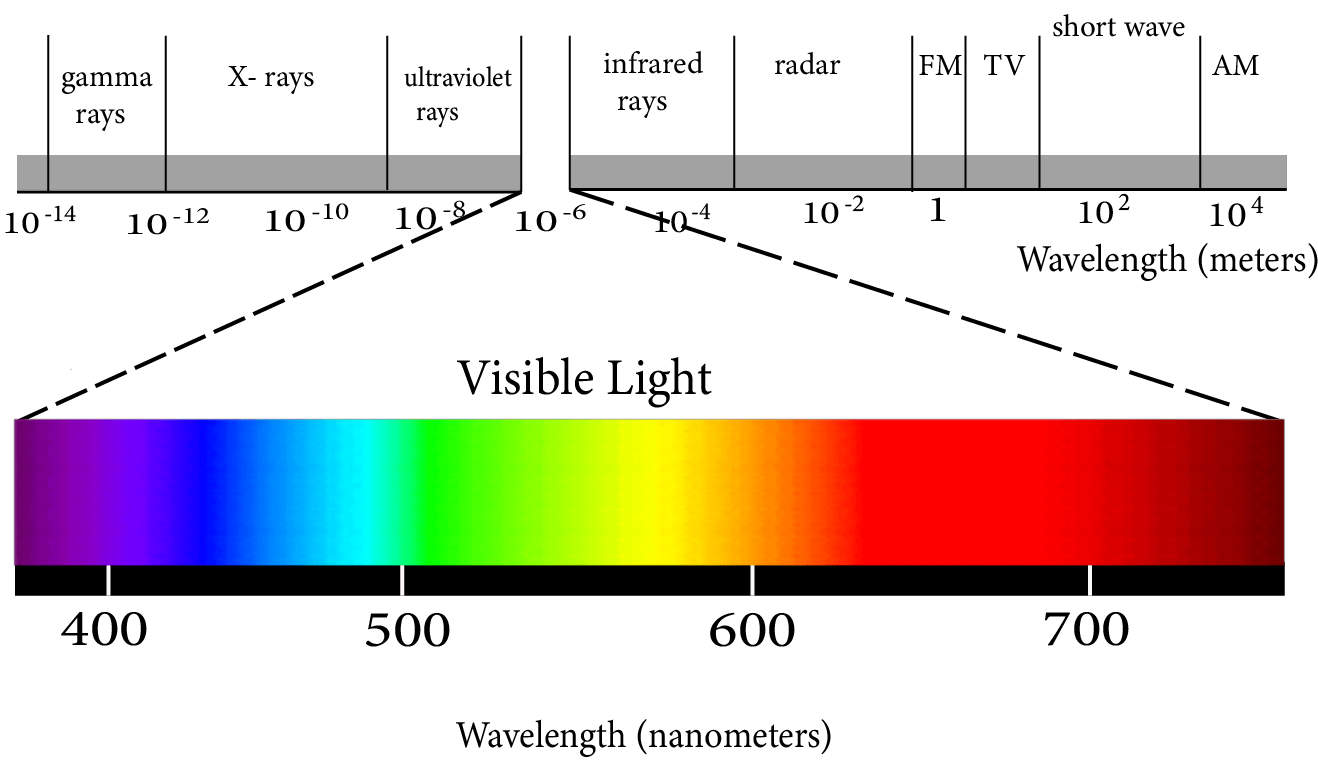(b) Particle Nature of Electromagnetic Radiation: Planck’s Quantum Theory

The essential points of this theory are:

(i) Energy is emitted or absorbed discontinuously in the form of small discrete packets of energy rather than constantly. A 'quantum' is the name given to each such energy packet. This quantum of energy is known as a photon in the case of light.

(ii) Only one photon can be delivered to one electron or other particles at a time.

(iii) It is impossible to divide or distribute a quantum.

(iv) The frequency of radiation is precisely proportional to the energy of each quantum.

E=hc/λ, where h = Planck's constant = 6.626 10-34Js

(v) A body's total energy emitted or absorbed will be quantified in whole numbers.

This is also known as "energy quantisation."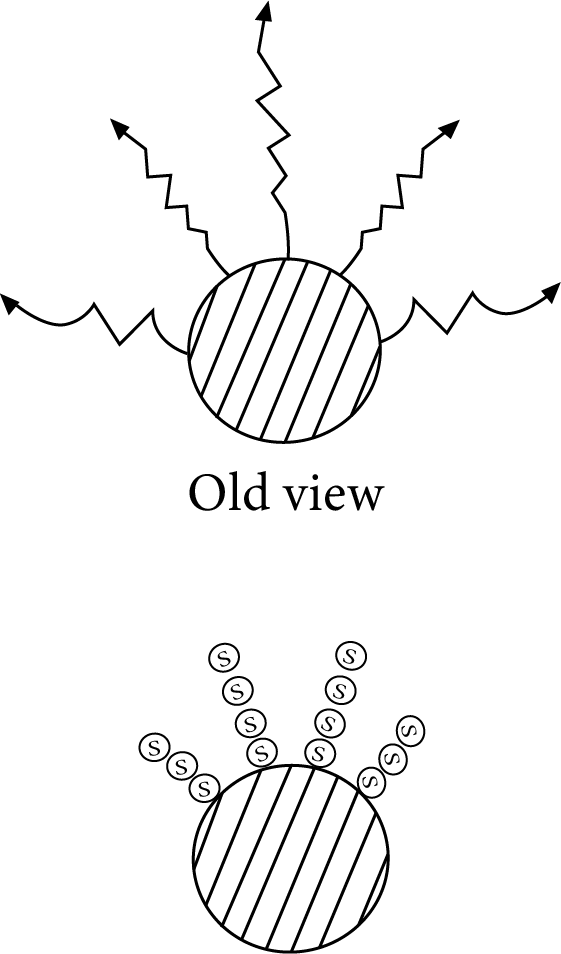(c)  Description of Black Body Radiation

• The energy emitted increases as the temperature rises, increasing the frequency of the emitted radiations. The wavelength moves to lower values as the frequency rises.

(d) Photoelectric Effect

• When a photon of a specific frequency strikes the surface of a metal atom, it imparts all of its energy to the metal atom's electron. The electron is ejected from the device, metal only if the photon's energy is sufficient to the force of attraction of the electron by the nucleus. As a result, photoelectrons are only expelled when The frequency of incident light has a minimum frequency (threshold). The Threshold energy required for emission is called “Work Function”.

• If the frequency of the incident light (ν) is more than the threshold frequency (ν0 ), the excess energy is imparted to the electron as kinetic energy. Hence,

• the Energy of one quantum = Threshold Energy + Kinetic Energy.

• When ν> ν0 , then on increasing the intensity the number of quanta incidents increases thereby increasing the number of photoelectrons ejected.

• When ν0 > ν, then on further increasing the frequency, the energy of each photon increases and thus the kinetic energy of each ejected electron increases.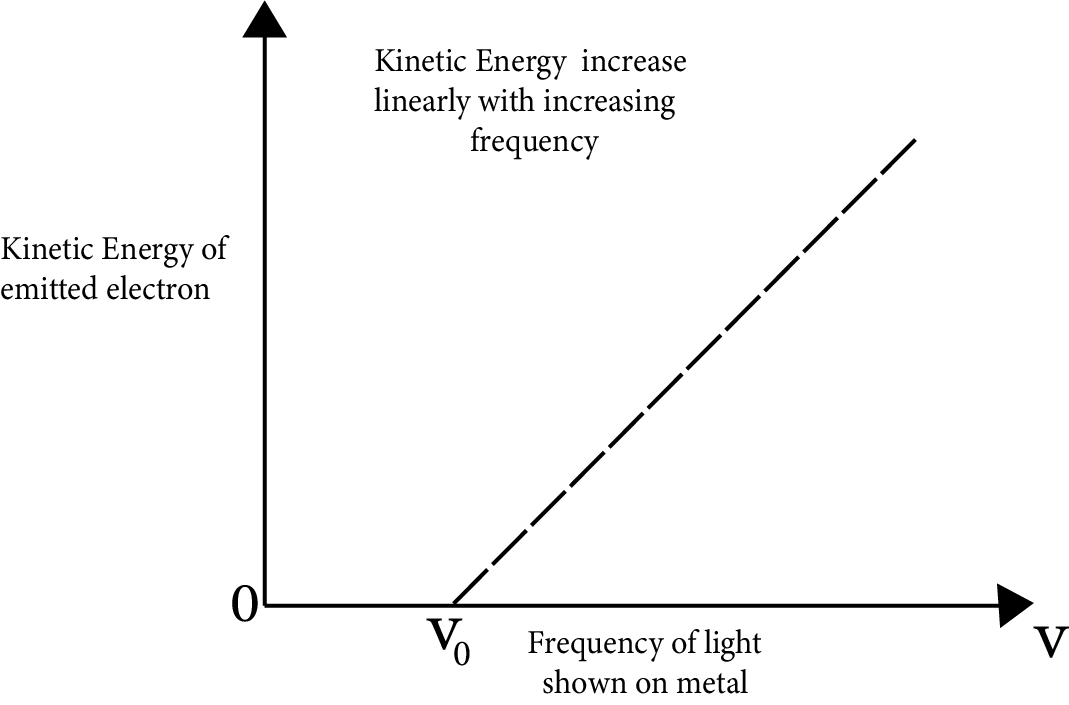(e) Evidence for the Quantized- Electronic Energy Levels: Atomic Spectra-

• When a photon of a specific frequency strikes the surface of a metal atom, it imparts all of its energy to the metal atom's electron. The electron is ejected from the device.  metal only if the photon's energy is sufficient to  the force of attraction of the electron is overcome by the nucleus. As a result, photoelectrons are only expelled when  The frequency of incident light has a minimum frequency (threshold).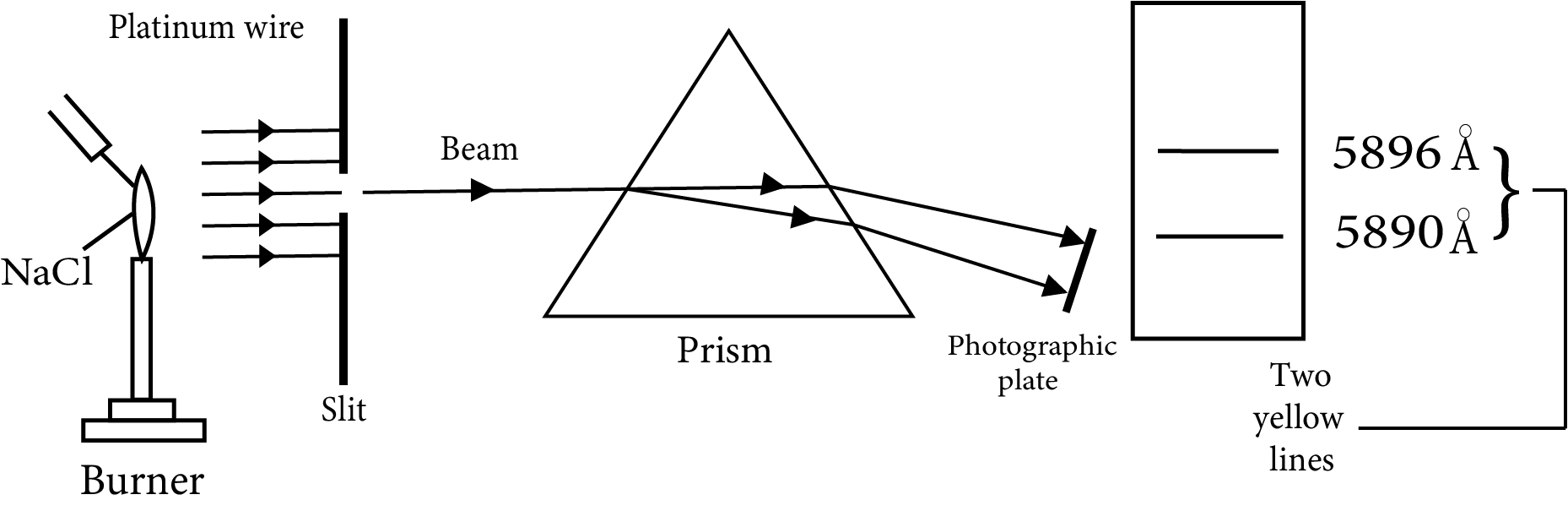• Atoms in the gas phase, on the other hand, do not have a continuous range of wavelengths from red to blue. Rather than emitting light at specific wavelengths, they emit light at certain wavelengths. Line spectra of atomic spectra are examples of such spectra.

Line Spectrum of Hydrogen

• When an electric current is transmitted across gaseous hydrogen, H2 is produced. When molecules dissociate, the energetically excited hydrogen atoms that result emit discrete frequency electromagnetic radiation. Several lines in the hydrogen spectra are named after their discoverer.

• Because the energy of an electron cannot change continuously but can only have fixed values, we get discrete lines rather than a continuous spectrum. As a result, the energy of one electron can be quantified.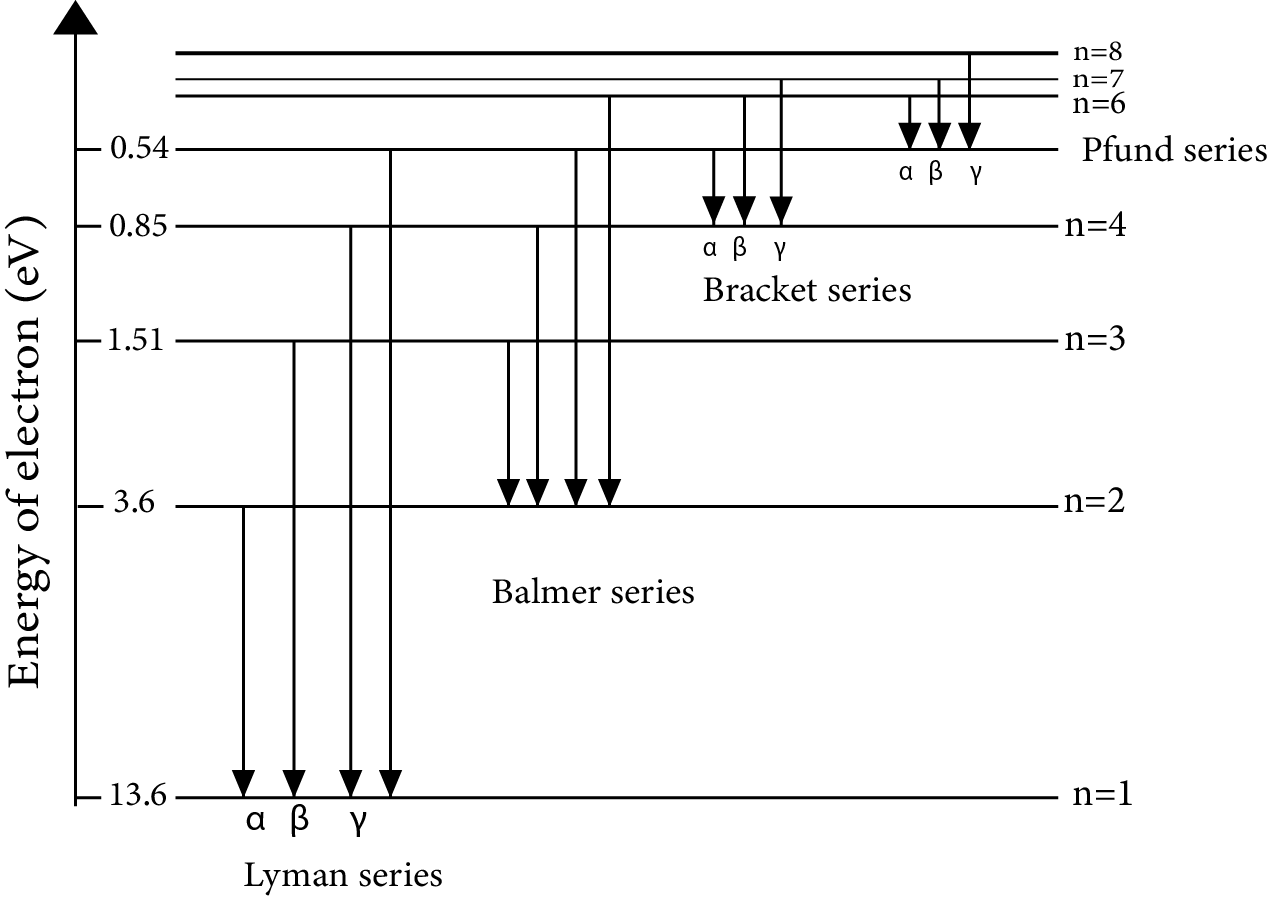### 4. Bohr's Model for Hydrogen Atom

• Neils Bohr (1913) was the first to quantitatively explain the general properties of the hydrogen atom's structure and spectrum. He applied Planck's concept of energy quantification.

• Postulates are given as-

(i) The electron in a hydrogen atom can move in a circular path around the nucleus with a defined radius and energy. Orbits, stationary states, and permitted energy states are all terms used to describe these pathways. Around the nucleus, these orbits are organised concentrically.

ii) An electron's energy in an orbit does not alter over time. When the requisite quantity of energy is absorbed by the electron or energy is emitted when the electron goes from a higher stationary state to a lower stationary state, the electron will move from a lower stationary state to a higher stationary state.

(iii) When a transition occurs between two stationary states that differ in energy by E, the frequency of radiation received or emitted is given by: v =  \$\dfrac{ΔE}{h}\$

(iv) The energies of the lower and higher permissible energy levels, respectively, are E1 and E2. Bohr's frequency rule is the name given to this equation.

iv) An electron's angular momentum is quantized. It can be represented as equation- mevr= \$\dfrac{nh}{2π}\$

where n = 1,2,3..... in a given stationary condition.

The hydrogen atom, according to Bohr's theory:

a) The electron's stationary states are numbered n = 1,2,3,..... Principal quantum numbers are these integral numbers.

b) The stationary state radii are written as rn = n2 a0

where a0 = 52.9 pm.

The first stationary state, known as the Bohr orbit, has a radius of 52.9 pm. The electron in a hydrogen atom is normally found in this orbit (n=1). The value of r will increase as n increases. To put it another way, the electron will be present outside of the nucleus.

b) The energy of the electron's stationary state is the most crucial property linked with it. The expression provides the answer.

En = \$\dfrac{-R_{H}}{n^{2}}\$

where RH is Rydberg’s constant and its value is 2.18 × 10–18 J.

En = \$\dfrac{− 2.18 × 10^{–18} Z^{2}}{n^{2}}~J\$

rn = \$\dfrac{52.9n^{2}}{Z}~pm\$

Transition of Electron

When electrons are absorbed or emitted, energy is absorbed or emitted.The electron excites or de-excites, as the case may be. The difference in energy between the two orbits is

ΔE= \$\dfrac{-R_{H}}{n_f^2}-\dfrac{-R_{H}}{n_i^2}\$

ΔE= \$-R_{H}(\dfrac{1}{n_f^2}-\dfrac{1}{n_i^2})~J/atom\$

Limitations of Bohr’s Model

• The following points were unable to account for using Bohr's model.

• (i)This model also fails to explain the spectrum of non-hydrogen atoms, such as the helium atom, which has only two electrons. Furthermore, Bohr's theory failed to explain spectral line splitting in the presence of a magnetic field (Zeeman effect) or an electric field (Stark effect).

• (ii) It could not account for atoms' ability to form molecules through chemical bonding.

### 5. Towards Quantum Mechanical Model of an Atom

Two important developments which contributed  in the formulation of such a model were :

(a) Dual Behaviour of Matter

According to de Broglie, matter should have dual behaviour, i.e. particle and wave-like qualities.

λ=h/mv

Every moving item, according to de Broglie, has a wave character. The wavelengths associated with ordinary objects are so short (because of their large masses) that their wave properties cannot be detected. Electrons and other subatomic particles (with very small masses) have wavelengths that can be measured experimentally.

(b) Heisenberg Uncertainty Principle

It asserts that determining the exact position and exact momentum (or velocity) of an electron at the same time is impossible. It can be expressed mathematically as an equation-

(Δx). (Δp) ≥ h/4π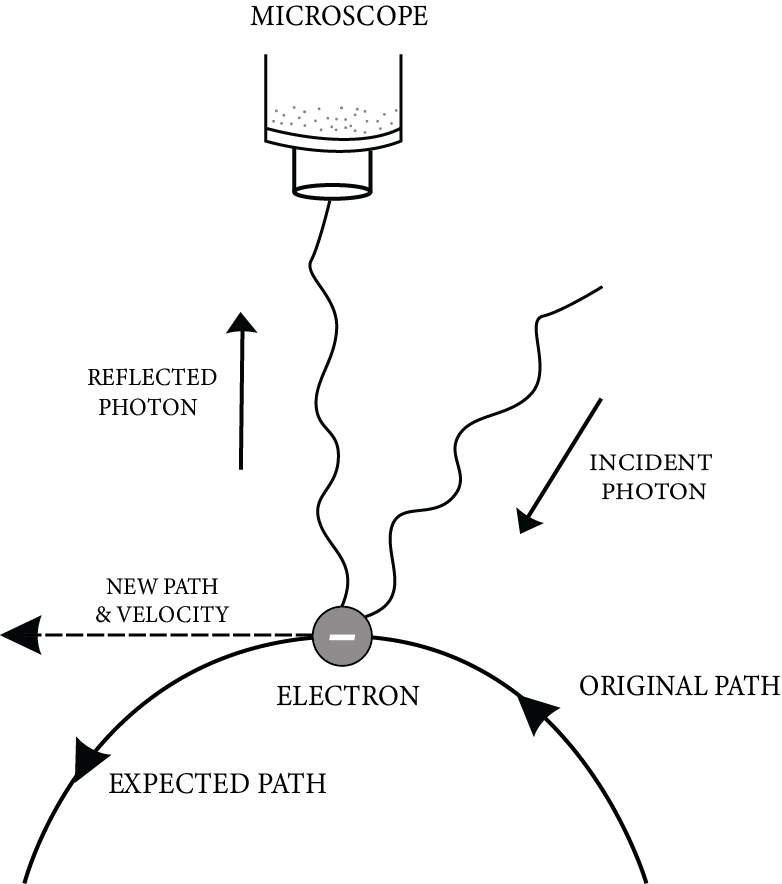### 6. Quantum Mechanical Model of an Atom

(A) Quantum Numbers-

(i) The Principal Quantum Number (n)

It indicates which shell an electron belongs to. n = 1,2,3,4,5….On the basis of electronic leaps between these shells, this number helps to describe the main lines of the spectrum.

(a) It offers the average distance between the nucleus and the electron. The distance from the nucleus increases as n increases.

(b) It determines the energy of a hydrogen atom or a hydrogen-like species fully. Only the value of n determines the energy of an H-atom or H-like species. Energy in ascending order: 1, 2, 3, 4, 5, and so on.

(c) The energy of multi-electron species is determined by both the primary and azimuthal quantum numbers.

(ii) Azimuthal Quantum Number (l):

Orbital Angular Momentum or Subsidiary Quantum Number. Because there are many subshells within a single shell, the number of electronic jumps increases, which explains the appearance of tiny lines in the spectrum. This quantum number indicates:

(a) the number of subshells in a shell;

(b) the number of subshells in a shell; and

(c) the number of subshells in a shell.

(d) An electron's angular momentum in a subshell.

 Value of l Designation of Subshell 0 s 1 p 2 d 3 f

(iii) Magnetic Quantum Number(m):

This quantum number is needed to explain why each spectral line divides into a number of lines when the source providing the line spectrum is placed in a magnetic field (Zeeman effect). The number of preferred electron orientations in a subshell is determined by the magnetic quantum number. Because each orientation corresponds to an orbital, the number of orbitals present in any subshell is determined by the magnetic quantum number. The value of m can be anything between – l and + l, including zero.

• The total possible values of m in a given subshell = 2l + 1

• Total no. of orbitals in a given shell = n2

(iv) Spin Quantum Number

The electron in an atom not only moves around the nucleus but also spins around its own axis, giving it a spin quantum number. Because an electron in an orbital can spin either clockwise or counterclockwise. As a result, s can only have two values. This quantum number, -1/2 or +1/2, is used to describe the magnetic characteristics of substances.

(B) Shapes of Atomic Orbitals

(I) s-orbitals have the following characteristics:

(a) They are non-directional and spherically symmetric, which means that the chance of finding an electron at a given distance is the same in all directions.

(b) The 1s and 2s orbitals have the same shape, but the 2s orbital is larger.

(c) A node is a spherical shell within the 2s orbital where the electron density is zero(II) P-orbitals have two lobes on either side of the plane that runs through the nucleus. The p-orbital is formed like a dumbbell. In p-orbitals, there are three distinct electron cloud orientations. As a result, the lobes of the p-orbital can be thought of as being along the x, y, and z axes. As a result, they are referred to as px, py, and pz. The three p-orbitals are aligned in the same direction at right angles to each other.(III) d-orbital shapes:

(a) They are denoted by the letters dxy, dyz, dzx, and dx2-y2. They resemble a four-leaf clover in appearance. The second d orbital, designated dz2, resembles a doughnut.

(b) In the absence of a magnetic field, all five d orbitals have the same energy.

(c) The d orbitals have a magnetic quantum number of –2, –1,0,+ 1,+ 2 and an azimuthal quantum number of l = 2.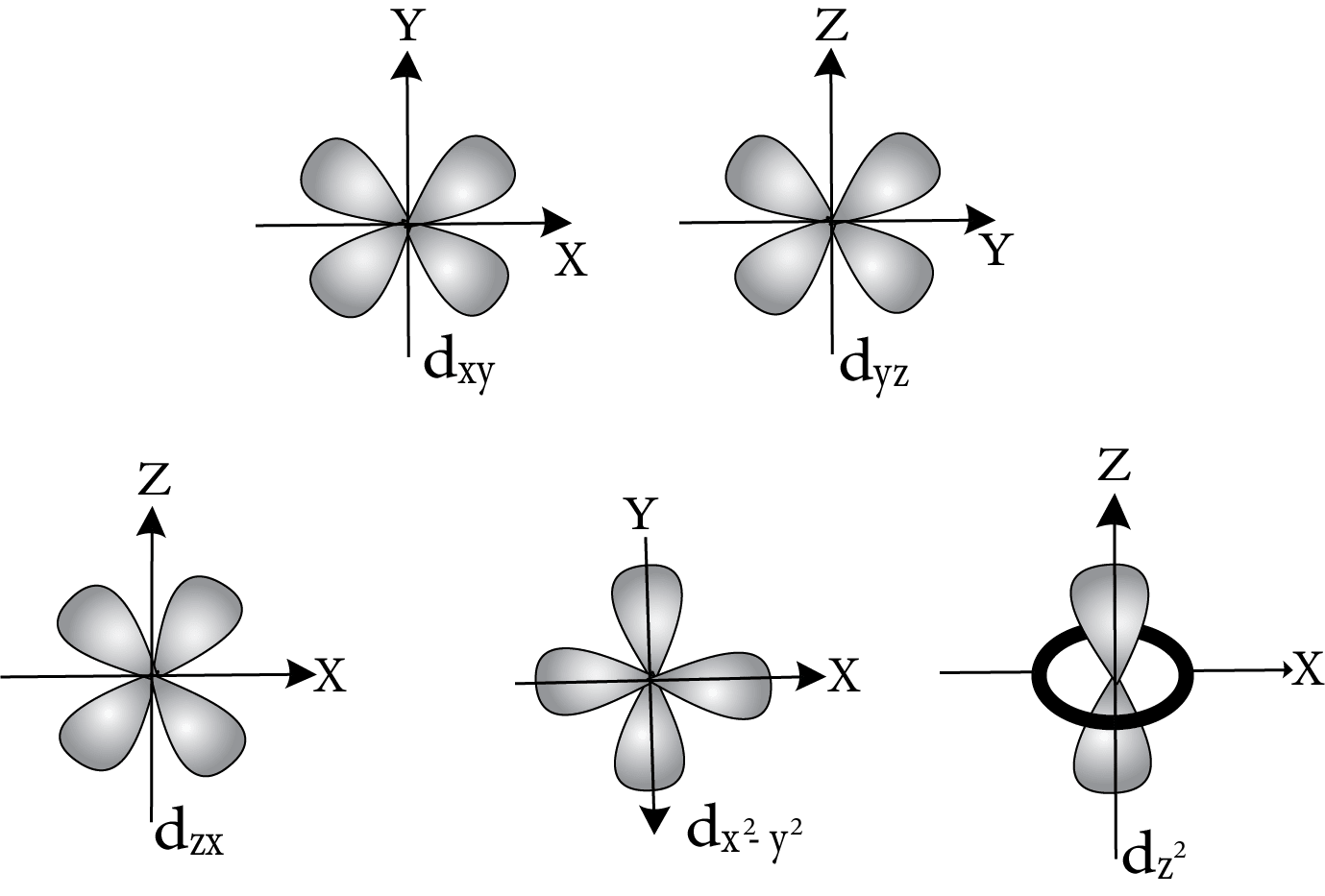Energies of Orbitals

In the absence of a magnetic field, the three p-orbitals of a specific main shell have the same energy.

Similarly, the energy of all five d-subshell orbitals of a given shell is the same.

Thus, the sequence of energy for the H-atom is:

1s< 2s = 2p <3s = 3p = 3d< 4s = 4p = 4d = 4f...........

The orbitals having the same energy are called degenerate.

Filling of Orbitals in Atom

The Aufbau Principle states that the orbitals of atoms are filled in order of increasing energy in their ground state. In other words, electrons occupy the lowest energy orbital initially and only enter higher energy orbitals once the lower energy orbitals have been filled.

Unlike the H-atom, where the energy of orbitals is solely determined by n, the energy of multi-electron orbitals is determined by both n and l. The (n+l) rule can be used to calculate their energy ranking.

The lower the value of (n+l) for an orbital, the lower its energy, according to this rule. If two types of orbitals have the same (n+l) value, the orbital with the lower n value has less energy.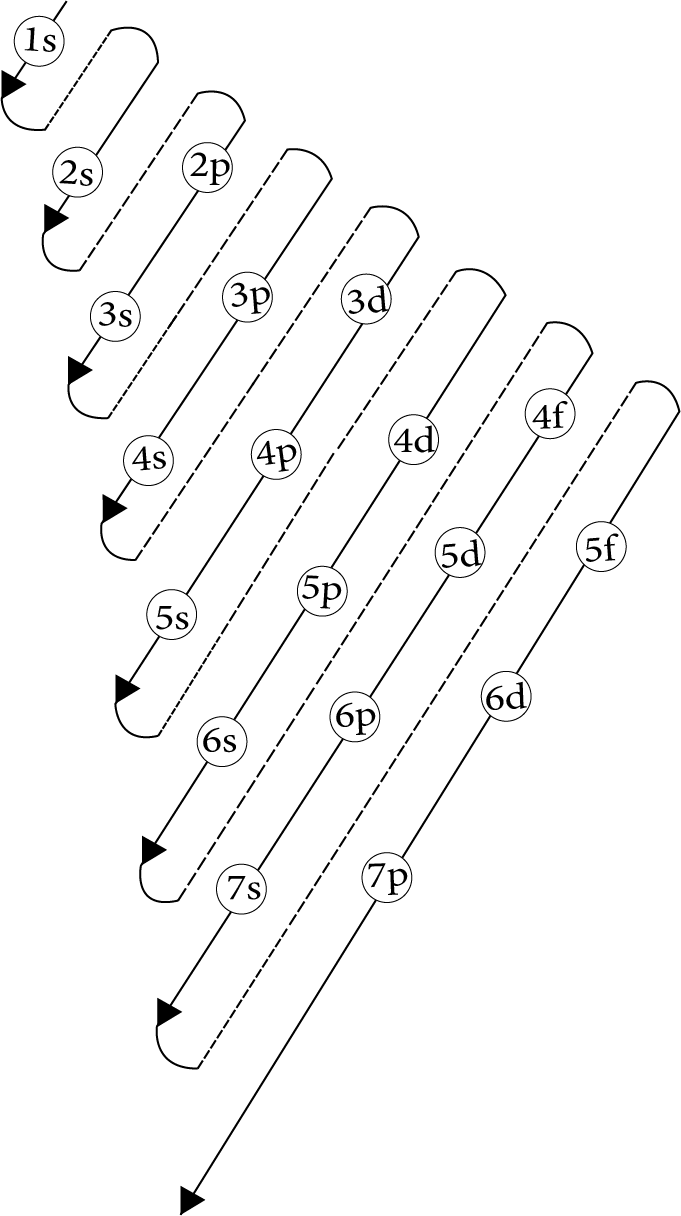Pauli Exclusion Principle:

An orbital can have a maximum of two electrons and these must have opposite spin.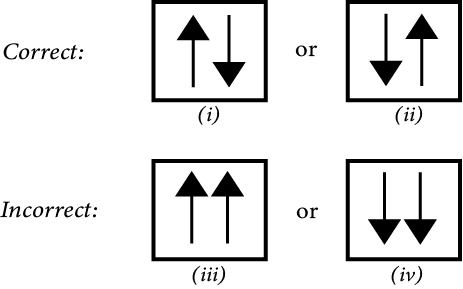Hund's Maximum Multiplicity Rule

It states that electron pairing in the p, d, and f orbitals is impossible until each orbital of a given subshell contains one electron. All singly occupied orbitals will have parallel spin as well.

Electronic Configuration of Atoms

The electronic configuration of an atom is the distribution of electrons into orbitals.

The electronic configurations of different atoms can be expressed quite easily if one remembers the basic laws that govern the filling of different atomic orbitals. The orbital picture of these elements can be represented as follows: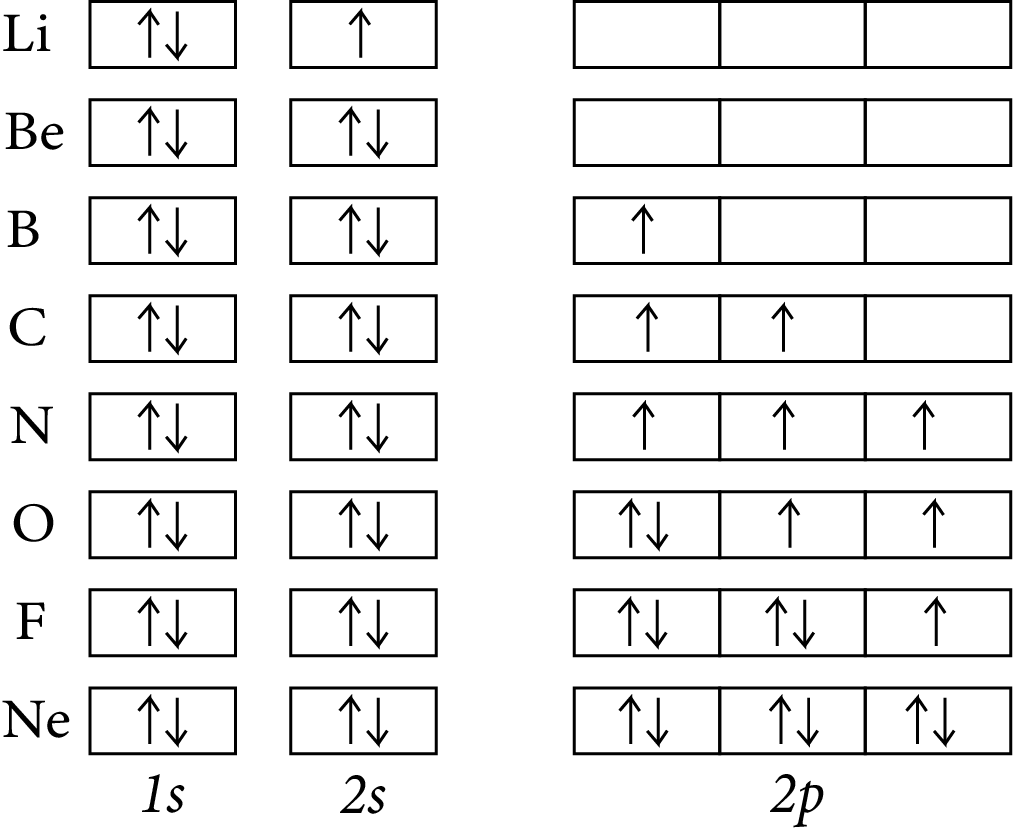### 7. Stability of Completely Filled and Half Filled Subshells

(a) Electron Distribution that is Symmetrical:

It is widely known that symmetry promotes stability. Subshells that are totally filled or half-filled have a symmetrical distribution of electrons and are thus more stable. The energy of electrons in the same subshell (here 3d) is the same, but their spatial distribution is different. As a result, their mutual shielding is minimal, and the electrons are more strongly drawn to the nucleus.

(b) Exchange Energy

When two or more electrons with the same spin are present in the degenerate orbitals of a subshell, the stabilising effect occurs. These electrons have a tendency to swap locations, and the energy produced as a result of this swap is known as exchange energy. When the subshell is half-filled or totally filled, the maximum number of exchanges possible is reached. As a result, the exchange of energy and stability are at their highest levels.

eg. for Cr : [Ar] 4s1 3d5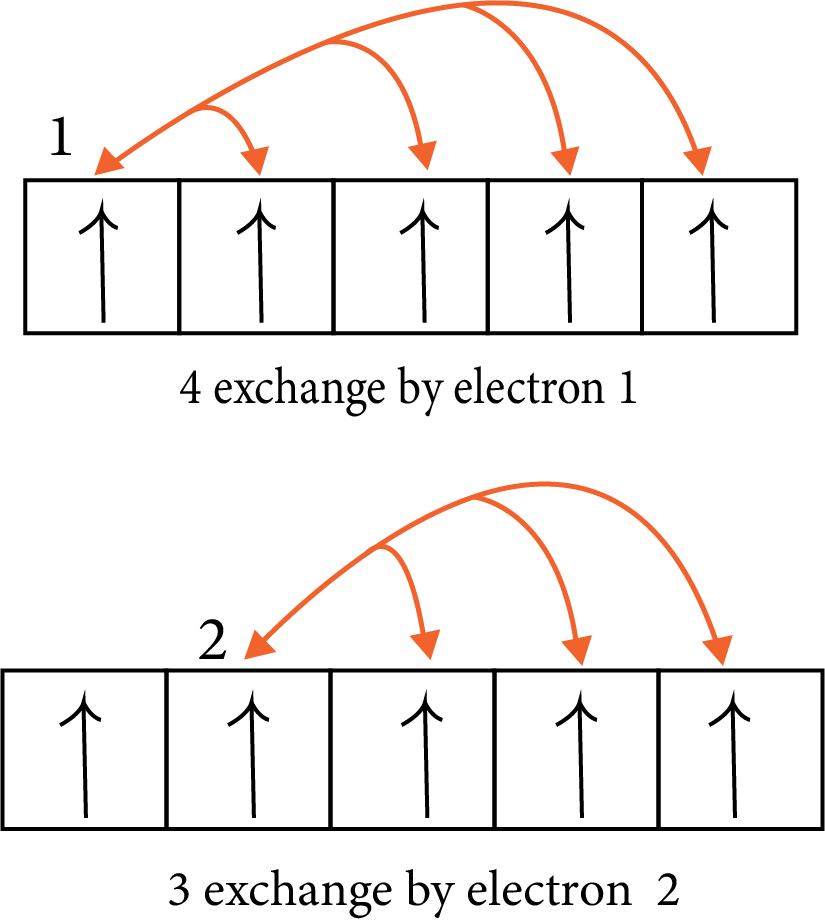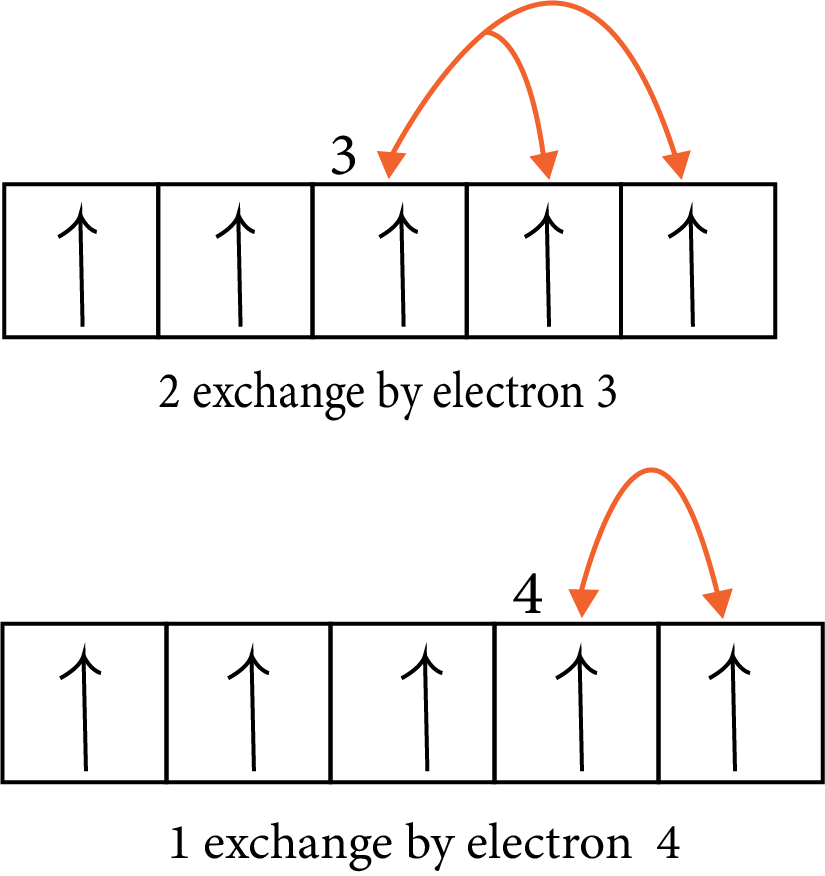### Solved Examples from the Chapter

Example-1. Explain the distinction between a particle and a wave.

Solution: (a) A particle has a well-defined position in space, i.e., it is localised in space, such as a grain of sand, a cricket ball, and so on.

(b) When one particle occupies a specific space, no other particle can occupy the same place at the same time. To put it another way, particles do not collide.

(c) A wave is spread out in space; for example, when a stone is thrown into a pond of water, the waves begin to move out in the form of concentric rings. Similarly, the speaker's voice is heard by everyone in the audience. As a result, a wave becomes delocalized in space.

Key Points to Remember- Nature of the particles are of two types one is particle nature and the other is wave nature.

Example-2. Is the Heisenberg principle satisfied by the Bohr model?

Solution: No, it eliminates Bohr's well-defined circular courses (orbits) or trajectories. Because it is impossible to precisely specify the position and velocity of a subatomic particle like an electron at any one time, it is impossible to discuss electron trajectories or well-defined circular orbits.

Key Points to Remember- Need to remember Bohr's Model and Heisenberg's Uncertainty principle.

### Solved Questions from The Previous Year Question Papers

Question 1: Which of the following statements in relation to the hydrogen atom is correct?

(a) 3s, 3p and 3d orbitals all have the same energy

(b) 3s and 3p orbitals are of lower energy than 3d orbital

(c) 3p orbital is lower in energy than 3d orbital

(d) 3s orbital is lower in energy than 3p orbital

Solution: A hydrogen atom has only one electron that has no shielding from other electrons, and therefore its energy level only depends on its distance away from the nucleus, which is dependent on its value of (n).

Therefore, the orbitals of a hydrogen atom increase energy as follows:

1s<2s=2p<3s=3p=3d<4s=4p=4d=4f<.....

Therefore, option (a) is the correct answer.

Trick: Need to remember the energy order of the orbitals.

Question 2: Which of the following parameters are not the same for all hydrogen-like atoms and ions in their ground state?

(b) Speed of electron

(c) Energy of the atom

(d) Orbital angular momentum of electron

Solution:  Radius of the orbit, speed of the electron, and energy of an atom depend on Z whereas orbital angular momentum is independent of Z. Hence, orbital angular momentum remains the same for all hydrogen-like atoms and ions in the ground state  (n=1).

Therefore, option (d) is the answer.

Trick: Need to remember the different parameters of the hydrogen-like species.

Question 3: Which of the following are the limitations of Bohr’s model ?

(a) It could not explain the intensities or the fine spectrum of the spectral lines.

(b) No justification was given for the principle of the quantization of angular momentum.

(c) It could not explain why atoms should combine to form bonds.

(d) It could not be applied to single-electron atoms

Solution: Limitations of Bohr's Theory:

I) It does not account for the spectra of atoms or ions with multiple electrons.

ii) The influence of a magnetic field (Zeeman effect) or an electric field (Stark effect) on the spectra of atoms or ions was not accounted for in Bohr's atomic model. When a spectrum's source is placed in a high magnetic or electric field, each spectral line divides into a number of lines, according to research. On the basis of Bohr's model, this observation could not be explained.

iii) De-Broglie proposed that electrons, like light, had two distinct personalities. It resembles a particle or a wave. The electron was only treated as a particle by Bohr.

Therefore, options (a,b,c)  are the correct answer.

Trick: Need to remember the Bohr's Model of an atom and its limitations.

### Practice Questions

Question 1: The de–Broglie wavelength associated with a material particle is

(a) Directly proportional to its energy

(b) Directly proportional to momentum

(c) Inversely proportional to its energy

(d) Inversely proportional to momentum

Answer: (b) Directly proportional to momentum.

Question 2: An electron jumps from 6th energy level to 3rd energy level in an H-atom, how many lines belong to the visible region?

(a) 1

(b) 2

(c) 3

(d) Zero

Question 3: Azimuthal quantum number for the last electron in Na atom is

(a) 1

(b) 0

(c) 2

(d) 3

### Conclusion

Atoms are the fundamental constituents of all elements. They are the tiniest components of a substance that react chemically. John Dalton proposed the first atomic theory in 1808, which saw the atom as the ultimate indivisible element of matter. At the conclusion of the nineteenth century, it was demonstrated experimentally that atoms are divisible and made up of three parts. Electrons, protons, and neutrons are the three fundamental particles. The discovery of subatomic particles. Various atomic models were proposed to describe the structure of the atom as a result of particles. Thomson proposed in 1898 that an atom is made up of a homogeneous sphere of positive electricity. It's a material having electrons incorporated into it. This model, in which the atom's mass is assumed to equal Rutherford's renowned alpha particle, revealed that this theory was incorrect.

## Important Related Links for JEE Main 2022-23

See More## JEE Main Important Dates

View all JEE Main Exam Dates
JEE Main 2023 January and April Session exam dates and revised schedule have been announced by the NTA. JEE Main 2023 January and April Session will now be conducted on 24-Jan-2023 to 31-Jan-2023 and 6-Apr-2023 to 12-Apr-2023, and the exam registration closes on 12-Jan-2023 and Apr-2023. You can check the complete schedule on our site. Furthermore, you can check JEE Main 2023 dates for application, admit card, exam, answer key, result, counselling, etc along with other relevant information.
See More
View all JEE Main Exam Dates## JEE Main Information

Application Form
Eligibility Criteria
Reservation Policy
Exam Centres
NTA has announced the JEE Main 2023 January session application form release date on the official website https://jeemain.nta.nic.in/. JEE Main 2023 January and April session Application Form is available on the official website for online registration. Besides JEE Main 2023 January and April session application form release date, learn about the application process, steps to fill the form, how to submit, exam date sheet etc online. Check our website for more details. April Session's details will be updated soon by NTA.## JEE Main Syllabus

View JEE Main Syllabus in Detail
It is crucial for the the engineering aspirants to know and download the JEE Main 2023 syllabus PDF for Maths, Physics and Chemistry. Check JEE Main 2023 syllabus here along with the best books and strategies to prepare for the entrance exam. Download the JEE Main 2023 syllabus consolidated as per the latest NTA guidelines from Vedantu for free.
See More
View JEE Main Syllabus in Detail## JEE Main 2023 Study Material

View all study material for JEE Main
JEE Main 2023 Study Materials: Strengthen your fundamentals with exhaustive JEE Main Study Materials. It covers the entire JEE Main syllabus, DPP, PYP with ample objective and subjective solved problems. Free download of JEE Main study material for Physics, Chemistry and Maths are available on our website so that students can gear up their preparation for JEE Main exam 2023 with Vedantu right on time.
See More
All
Mathematics
Physics
Chemistry
See All## JEE Main Question Papers

see all
Download JEE Main Question Papers & ​Answer Keys of 2022, 2021, 2020, 2019, 2018 and 2017 PDFs. JEE Main Question Paper are provided language-wise along with their answer keys. We also offer JEE Main Sample Question Papers with Answer Keys for Physics, Chemistry and Maths solved by our expert teachers on Vedantu. Downloading the JEE Main Sample Question Papers with solutions will help the engineering aspirants to score high marks in the JEE Main examinations.
See MoreView all JEE Main Important Books
In order to prepare for JEE Main 2023, candidates should know the list of important books i.e. RD Sharma Solutions, NCERT Solutions, RS Aggarwal Solutions, HC Verma books and RS Aggarwal Solutions. They will find the high quality readymade solutions of these books on Vedantu. These books will help them in order to prepare well for the JEE Main 2023 exam so that they can grab the top rank in the all India entrance exam.
See More
Maths
NCERT Book for Class 12 Maths
Physics
NCERT Book for Class 12 Physics
Chemistry
NCERT Book for Class 12 Chemistry
Physics
H. C. Verma Solutions
Maths
R. D. Sharma Solutions
Maths
R.S. Aggarwal Solutions
See All## JEE Main Mock Tests

View all mock tests
JEE Main 2023 free online mock test series for exam preparation are available on the Vedantu website for free download. Practising these mock test papers of Physics, Chemistry and Maths prepared by expert teachers at Vedantu will help you to boost your confidence to face the JEE Main 2023 examination without any worries. The JEE Main test series for Physics, Chemistry and Maths that is based on the latest syllabus of JEE Main and also the Previous Year Question Papers.
See More## JEE Main 2023 Cut-Off

JEE Main Cut Off
NTA is responsible for the release of the JEE Main 2023 January and April Session cut off score. The qualifying percentile score might remain the same for different categories. According to the latest trends, the expected cut off mark for JEE Main 2023 January and April Session is 50% for general category candidates, 45% for physically challenged candidates, and 40% for candidates from reserved categories. For the general category, JEE Main qualifying marks for 2021 ranged from 87.8992241 for general-category, while for OBC/SC/ST categories, they ranged from 68.0234447 for OBC, 46.8825338 for SC and 34.6728999 for ST category.
See More## JEE Main 2023 Results

NTA will release the JEE Main 2023 January and April sessions exam dates on the official website, i.e. {official-website}. Candidates can directly check the date sheet on the official website or https://jeemain.nta.nic.in/. JEE Main 2023 January and April sessions is expected to be held in February and May. Visit our website to keep updates of the respective important events of the national entrance exam.
See More
Rank List
Counselling
Cutoff
JEE Main 2023 state rank lists will be released by the state counselling committees for admissions to the 85% state quota and to all seats in IIT colleges. JEE Main 2023 state rank lists are based on the marks obtained in entrance exams. Candidates can check the JEE Main 2023 state rank list on the official website or on our site.## JEE Top Colleges

View all JEE Main 2023 Top Colleges
Want to know which Engineering colleges in India accept the JEE Main 2023 scores for admission to Engineering? Find the list of Engineering colleges accepting JEE Main scores in India, compiled by Vedantu. There are 1622 Colleges that are accepting JEE Main. Also find more details on Fees, Ranking, Admission, and Placement.
See More## FAQs on JEE Important Chapter - Atomic Structure

FAQ

1. What are some atomic structure facts?

Subatomic particles, which make up an atom, are made up of smaller particles. Electrons, protons, and neutrons are the subatomic particles in question. There are over 100 different types of atoms, 92 of which are natural and eight of which are man-made. The number of electrons, protons, and neutrons determines the type of atom.

2. What percentage of an atom is empty?

When you consider that atoms are 99 percent empty space and that they make up 100 percent of the universe, it's easy to realise that you're formed of nothing. Every human on the earth is made up of millions of atoms, all of which are 99 percent empty.

3. Is everything made up of atoms?

Everything in the cosmos is constituted of matter (excluding energy), and hence everything in the universe is formed of atoms. Protons, neutrons, and electrons are the three subatomic particles that make up an atom.## Notice board

JEE News
JEE Blogs